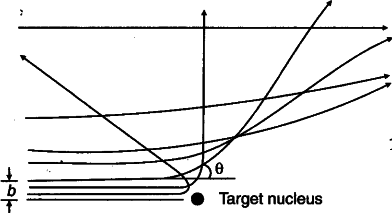# In the study of Geiger-Marseden experiment

In the study of Geiger-Marseden experiment onscattering of a.-particles by a thin foil of gold, draw I the trajectory of a-particles in the Coulomb field of target nucleus. Explain briefly how one gets the information on the size of the nucleus from this study.
From the relation $R={{R}_{0}}{{A}^{\frac{1}{3}}}$, where Ro is constant and A is the mass number of the nucleus, Show I that nuclear matter density is independent of A.R={ { R }_{ 0 } }{ { A }^{ \frac { 1 }{ 3 } } }
Density=\frac { mass }{ volume }
=\frac { mA }{ \frac { 4 }{ 3 } \pi { R }^{ 3 } } where;
m : Mass of one nucleon
A : Mass number
=\frac { mA }{ \frac { 4 }{ 3 } \pi R{ { \left( { { R }_{ 0 } }{ { A }^{ \frac { 1 }{ 3 } } } \right) }^{ 3 } } }
\frac { 3m }{ 4\pi { { R }_{ 0 } }^{ 3 } }
\Rightarrow Nuclear matter density is independent of A.